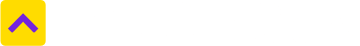# Convert Square Meter to Square Feet

One Square Meter is equal to 10.76391042 Square Feet
Select State
Select from unit
Square Meter
Select to unit
10.76391042
Square Feet

A unit of area measurement, square metre or square meter is used all across the world to measure two-dimensional spaces such as a field or floor and is globally accepted as the standard international unit of area. This is why this measurement unit is used in real estate quite often. The knowledge of the unit and the conversion process would thus come handy while buying property or flooring purposes, among other things.

A square metre, which is known often abbreviated as sq.m. or M2, is a unit of area measurement equal to a square measuring one meter on each side. By that calculation, it would take nearly 12 square meters to create the parking space for an average car.

While measuring real estate, it should be duly noted that the area is proportional to the square of the linear dimension. So, if the linear dimensions are doubled, the area assumes four times larger.

Since square meters are a unit that measures area, these are typically avoided when measuring length or distance in one direction. This precisely whey one can convert square metre into square foot. A square meter, for example, is equal to 10.76 square feet. However, square meter, also called the meter squared, can’t be converted to feet. However, when converting between square meters and areas such as square inches or square miles, the linear-unit conversion factor must be squared, if the conversion has to be done.

Even though square meter is globally used as a measurement unit, it is not popular in the United States.

### What are the most common land measurement units in India?

Hectares, acres, square meters and square yards are some of the common land measurement units used across India. While Bigha and Marla are commonly used in the North, terms such as Cent, Guntha and Ground belong to the South. Depending upon the names of the units, their sizes also vary from State to State.

### How much is Square Feet in Square Meter terms?

One Square Feet is equal to 0.09290303997 Square Meter. Click here to convert

### How much is Square Meter in Square Feet terms?

One Square Meter is equal to 10.76391042 Square Feet.
Trusted by
Partners© 2012-21 Locon Solutions Pvt. Ltd.